Gallery of examples

Next: More about the Up: Some planar isospectral domains Previous: Transplantation

# Gallery of examples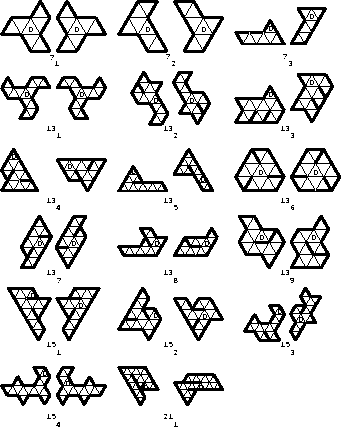Figure 4: Isospectral domains.

Figure 4 shows pairs of diagrams representing domains whose isospectrality can be verified using the method of transplantation. Each pair of diagrams represents not a single pair of isospectral domains, but a whole family of pairs of isospectral domains, gotten by replacing the equilateral triangles with general triangles so that the triangles labelled 0 are mapped onto one another by a translation and the remaining triangles are obtained from these by the appropriate sequence of reflections. We have seen two examples of this already, in Figures 3 and 1. Further examples generated in this way are shown in Section 5.

The pair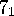is the pair of propeller diagrams discussed in detail above. The pair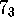yields a simplified version of the pair of isospectral domains given by Gordon, Webb, and Wolpert , , which was obtained by bisecting a pair of flat but non-planar isospectral domains given earlier by Buser . The pair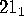yields the homophonic domains shown in Figure 1 above. In this case we must be careful to choose the relevant angle of our generating triangle to be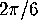since six of these angles meet around a vertex in each domain. If we do not choose the angle to be, then instead of planar domains we get a pair of isospectral cone-manifolds.

Note that in order for the pair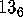to yield a pair of non-overlapping non-congruent domains we must decrease all three angles simultaneously, which we can do by using hyperbolic triangles in place of Euclidean triangles. Using hyperbolic triangles, we can easily produce isospectral pairs of convex domains in the hyperbolic plane, but we do not know of any such pairs in the Euclidean plane.

Next: More about the Up: Some planar isospectral domains Previous: Transplantation

Peter Doyle